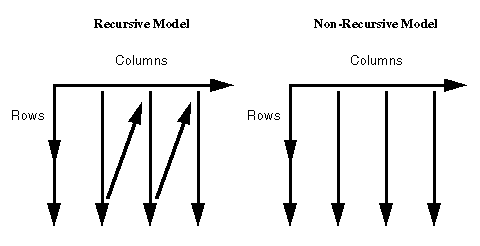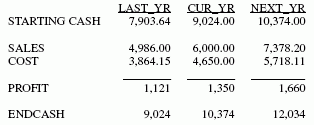Creating a Recursive Model

Models involving different time periods often require using the ending value of one time period as the starting value for the next. The calculations describing these situations have two characteristics:

• The labels on one or more RECAP rows are duplicates of other rows. They are used repeatedly to recompute certain values.
• A calculation may refer to a label not yet described, but provided later in the model. If, at the end of the model, a label that is needed is missing, a message is displayed.

Recursive models require that the columns are produced in sequential order, one by one. In nonrecursive models, all of the columns can be produced simultaneously. Schematically, these patterns are shown as follows.FML automatically switches to sequential order as soon as either of the two modeling conditions requiring the switch is recognized (either reuse of labels by different rows, or forward reference to a label in a calculation).

 Top of page
Example: Creating a Recursive Model

The following example illustrates recursive models. Note that one year of ENDCASH becomes the next year of STARTING CASH.

```DEFINE FILE REGION
CUR_YR=E_ACTUAL;
LAST_YR=.831*CUR_YR;
NEXT_YR=1.2297*CUR_YR;
END

TABLE FILE REGION
SUM LAST_YR CUR_YR NEXT_YR
FOR ACCOUNT
10\$\$ AS 'STARTING CASH' LABEL STCASH        OVER
RECAP STCASH(2,*) = ENDCASH(*-1);           OVER
" "                                         OVER
3000 AS 'SALES' LABEL SLS                   OVER
3100 AS 'COST' LABEL COST                   OVER
BAR                                         OVER
RECAP PROFIT/I5C = SLS - COST;              OVER
" "                                         OVER
RECAP ENDCASH/I5C = STCASH + PROFIT;
END```

The output is shown as follows.WebFOCUS